# Selina Solutions Concise Mathematics Class 6 Chapter 1: Number System Exercise 1(B)

Exercise 1(B) of Class 6 Mathematics covers the concepts of operations on larger numbers, along with their verification. Students can go through these solutions designed by experts in order to boost their exam preparation. They are very helpful to students who find difficulties in solving the problems of the Selina textbook. Practising these solutions particularly helps those students who failed to clear their doubts during class hours. PDF of Selina Solutions Concise Mathematics Class 6 Chapter 1 Number System Exercise 1(B) is free to download, from the links mentioned below

## Selina Solutions Concise Mathematics Class 6 Chapter 1 Number System Exercise 1(B) Download PDF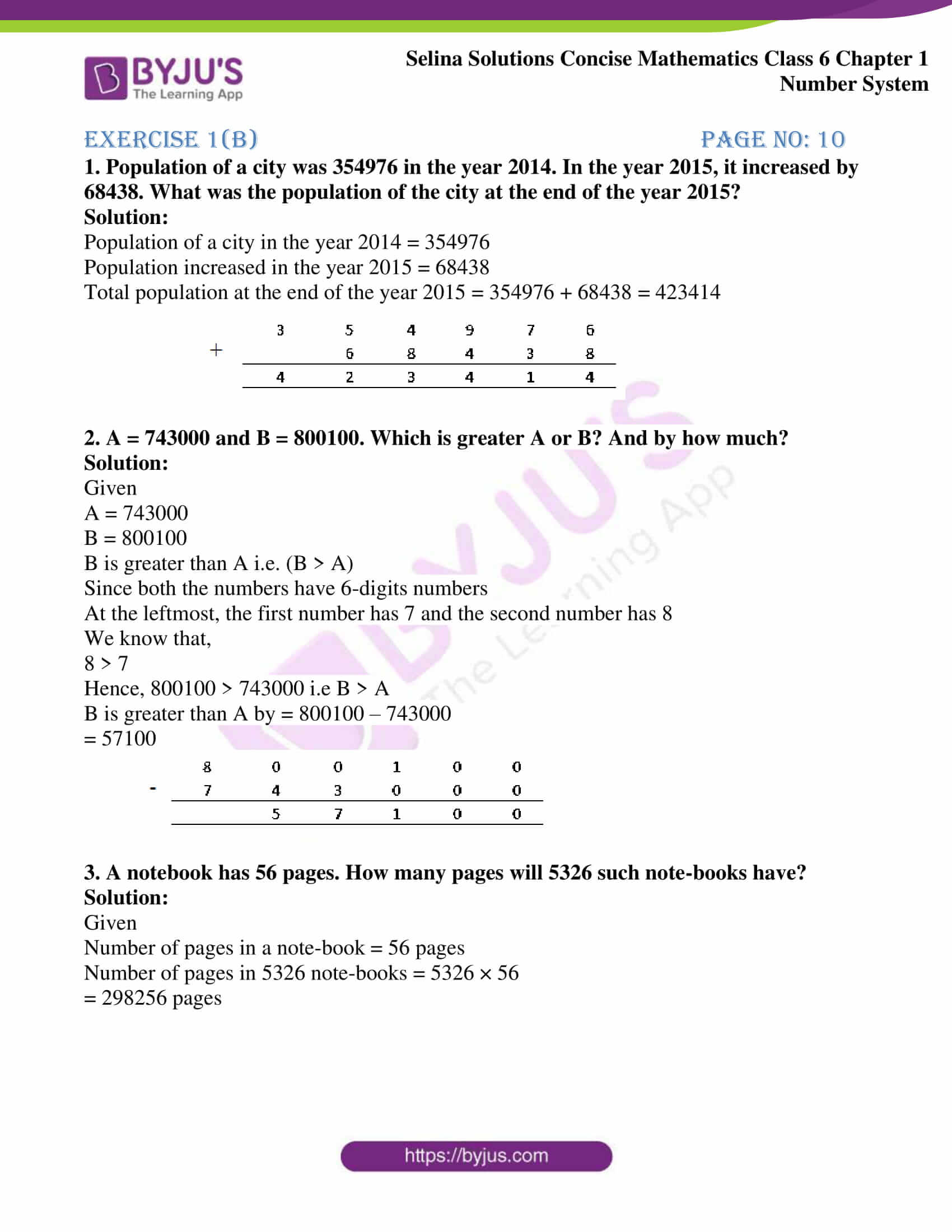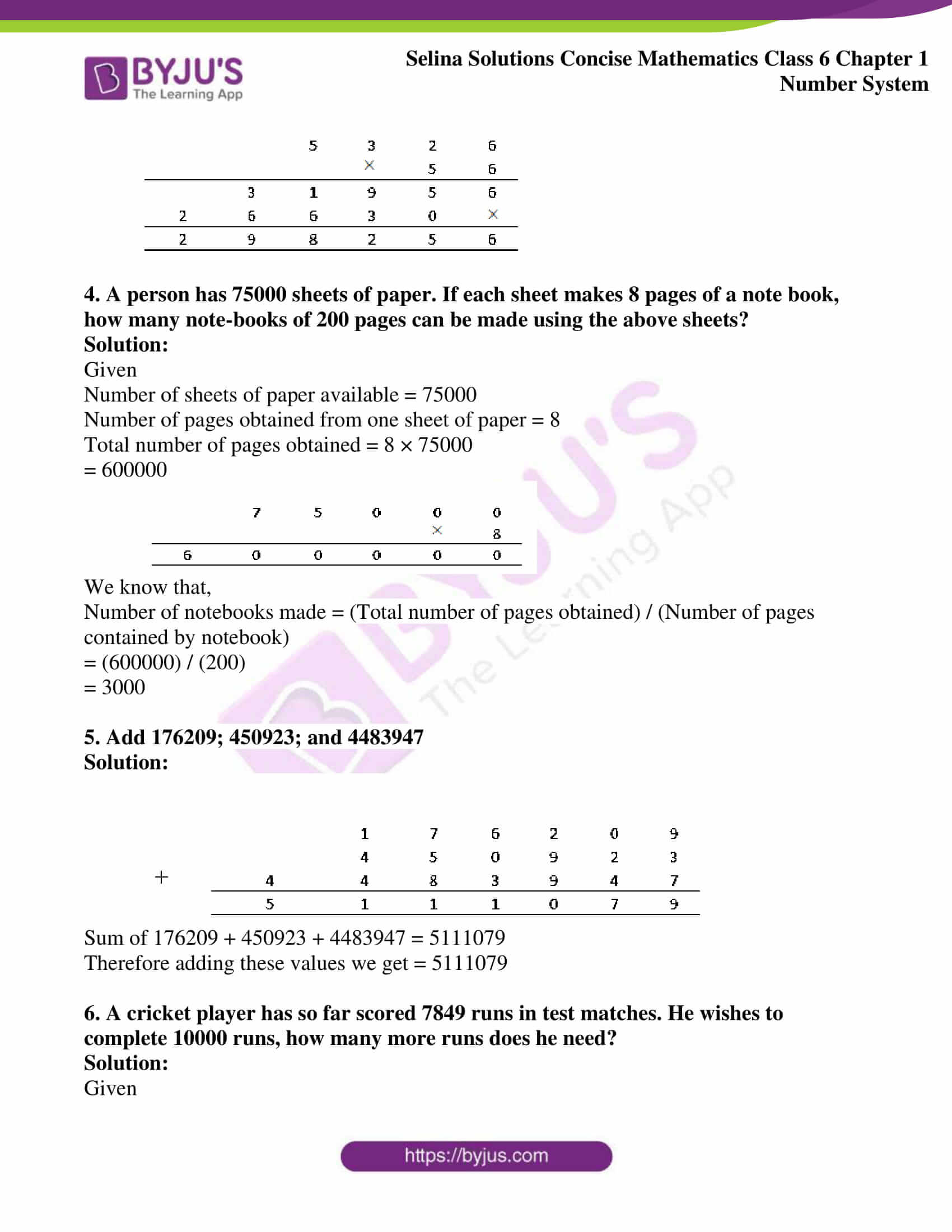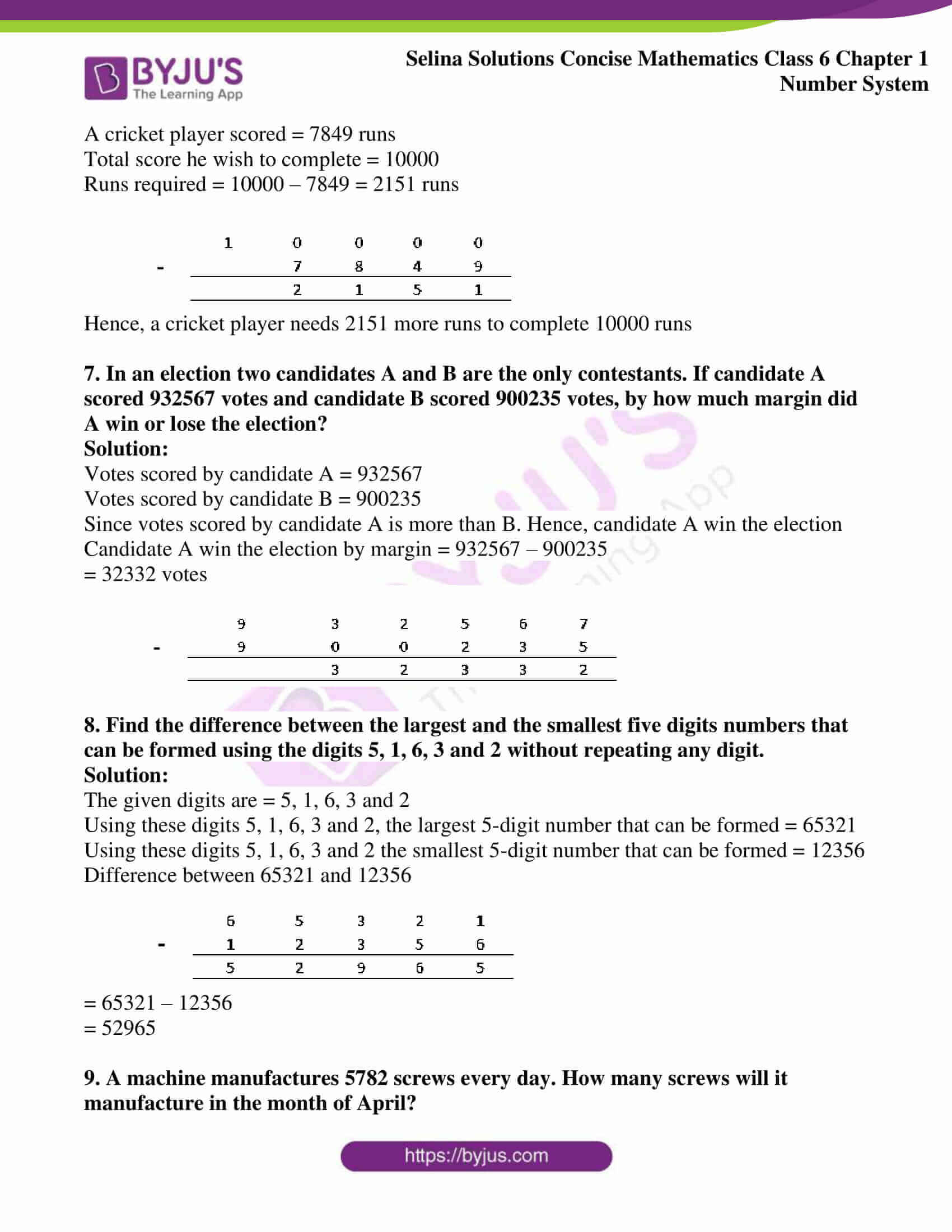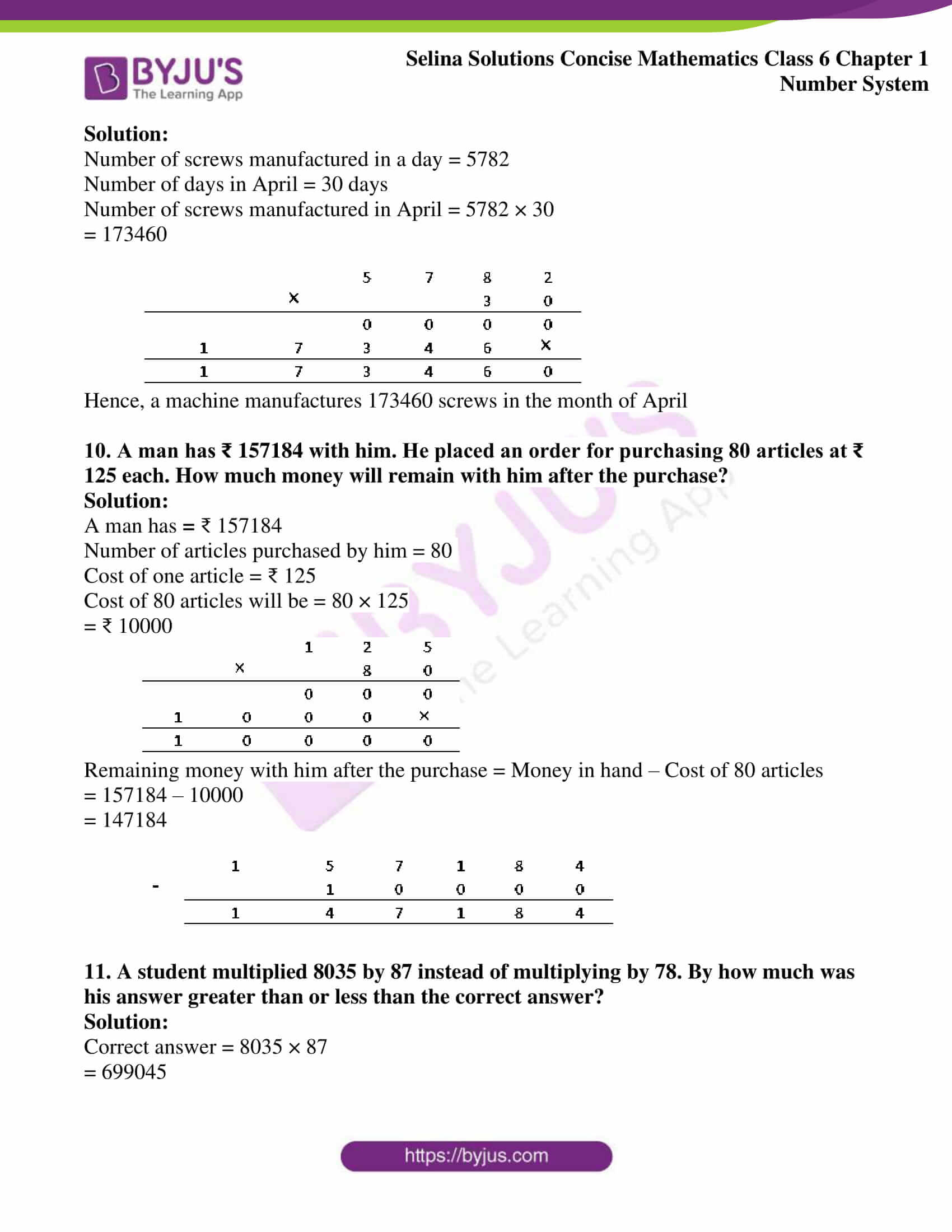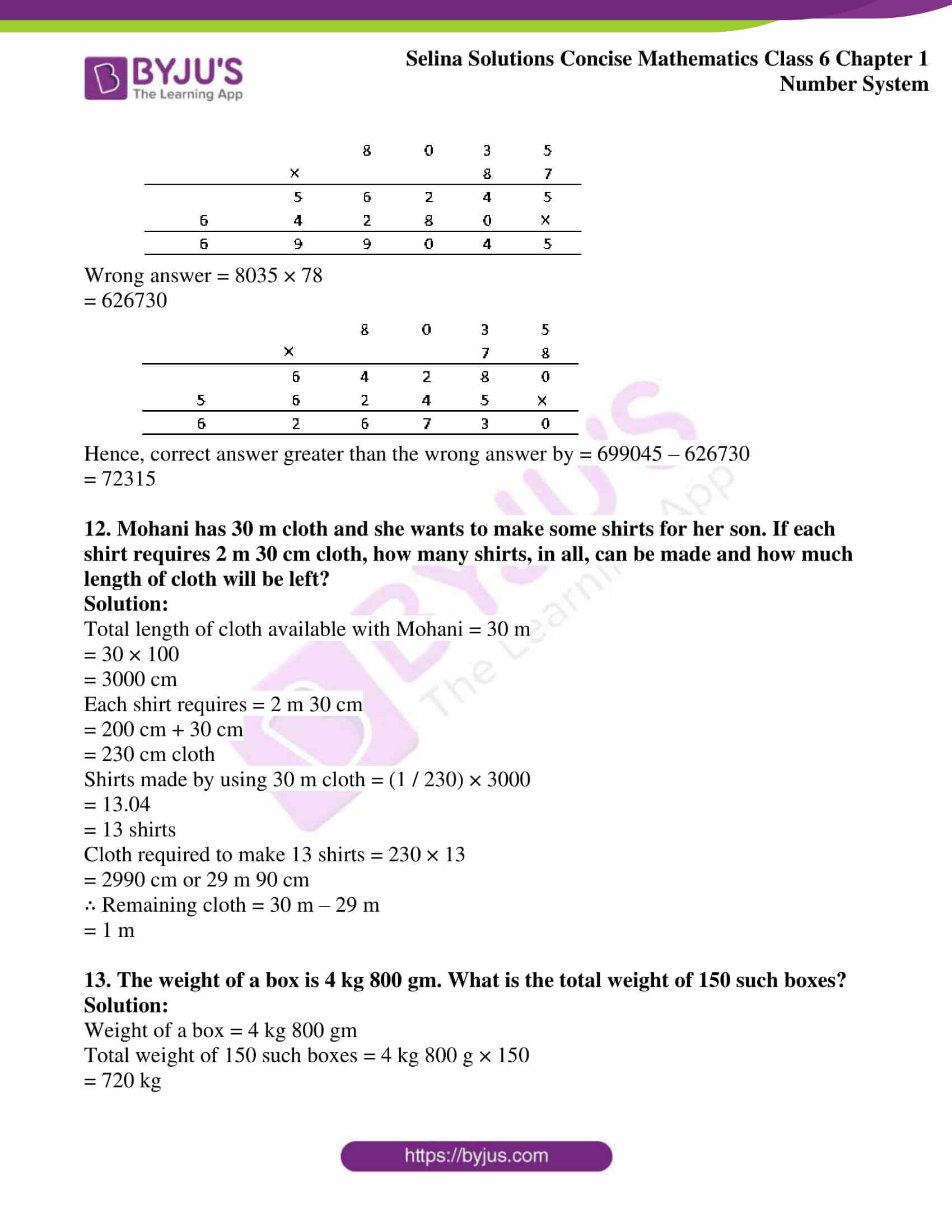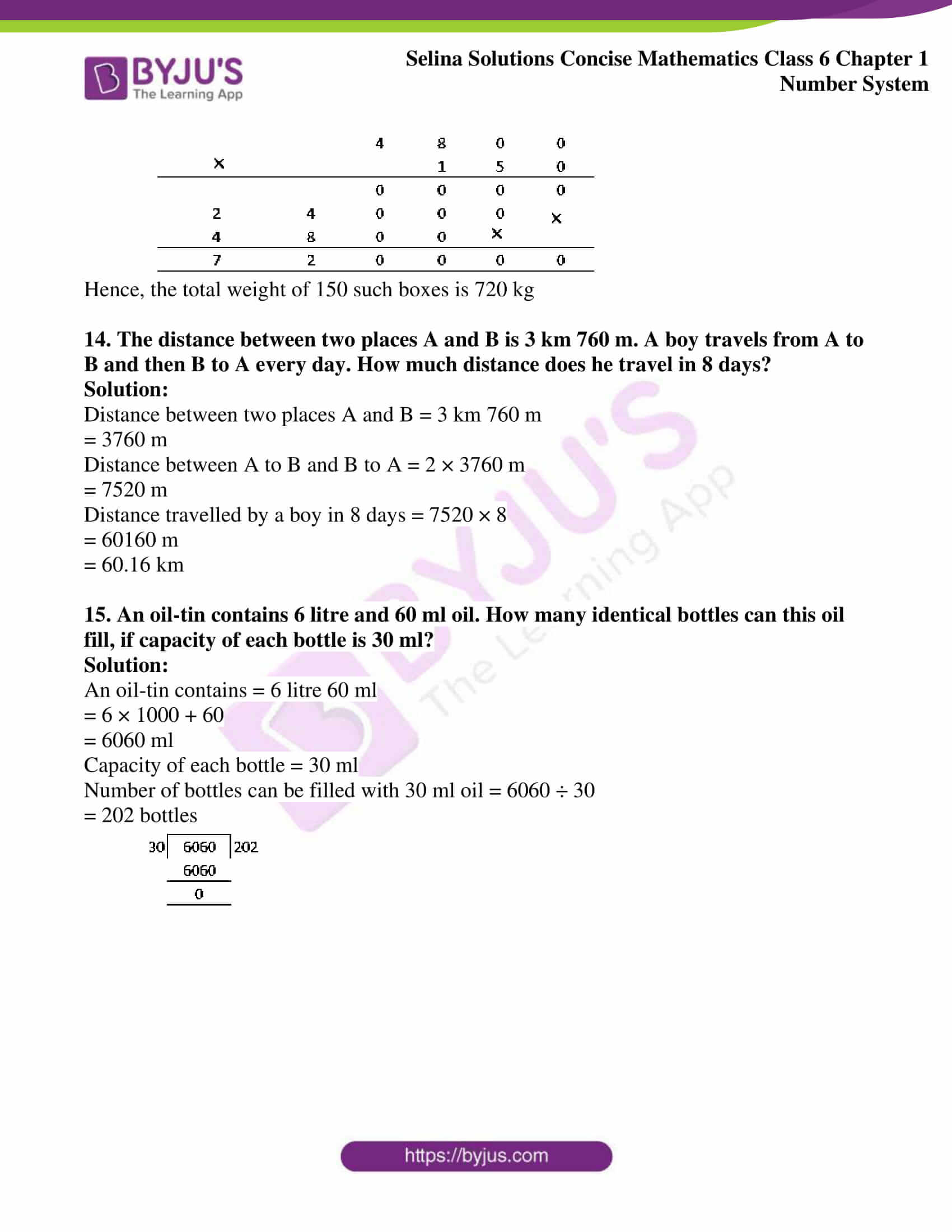### Access answers of Selina Solutions Concise Mathematics Class 6 Chapter 1 Number System

#### Exercise 1(B) page no: 10

1. Population of a city was 354976 in the year 2014. In the year 2015, it increased by 68438. What was the population of the city at the end of the year 2015?

Solution:

Population of a city in the year 2014 = 354976

Population increased in the year 2015 = 68438

Total population at the end of the year 2015 = 354976 + 68438 = 423414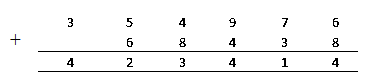2. A = 743000 and B = 800100. Which is greater A or B? And by how much?

Solution:

Given

A = 743000

B = 800100

B is greater than A i.e. (B > A)

Since both the numbers have 6-digits numbers

At the leftmost, the first number has 7 and the second number has 8

We know that,

8 > 7

Hence, 800100 > 743000 i.e B > A

B is greater than A by = 800100 – 743000

= 57100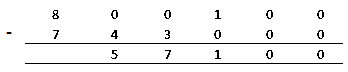3. A notebook has 56 pages. How many pages will 5326 such note-books have?

Solution:

Given

Number of pages in a note-book = 56 pages

Number of pages in 5326 note-books = 5326 × 56

= 298256 pages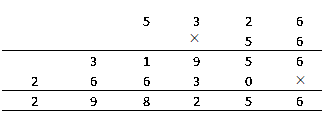4. A person has 75000 sheets of paper. If each sheet makes 8 pages of a note book, how many note-books of 200 pages can be made using the above sheets?

Solution:

Given

Number of sheets of paper available = 75000

Number of pages obtained from one sheet of paper = 8

Total number of pages obtained = 8 × 75000

= 600000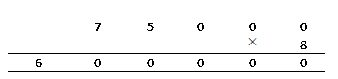We know that,

Number of notebooks made = (Total number of pages obtained) / (Number of pages contained by notebook)

= (600000) / (200)

= 3000

5. Add 176209; 450923; and 4483947

Solution: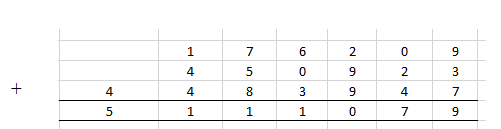Sum of 176209 + 450923 + 4483947 = 5111079

Therefore adding these values we get = 5111079

6. A cricket player has so far scored 7849 runs in test matches. He wishes to complete 10000 runs, how many more runs does he need?

Solution:

Given

A cricket player scored = 7849 runs

Total score he wish to complete = 10000

Runs required = 10000 – 7849 = 2151 runs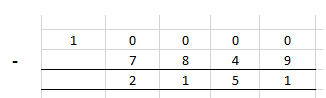Hence, a cricket player needs 2151 more runs to complete 10000 runs

7. In an election two candidates A and B are the only contestants. If candidate A scored 932567 votes and candidate B scored 900235 votes, by how much margin did A win or lose the election?

Solution:

Votes scored by candidate A = 932567

Votes scored by candidate B = 900235

Since votes scored by candidate A is more than B. Hence, candidate A win the election

Candidate A win the election by margin = 932567 – 900235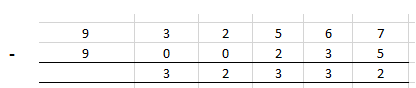8. Find the difference between the largest and the smallest five digits numbers that can be formed using the digits 5, 1, 6, 3 and 2 without repeating any digit.

Solution:

The given digits are = 5, 1, 6, 3 and 2

Using these digits 5, 1, 6, 3 and 2, the largest 5-digit number that can be formed = 65321

Using these digits 5, 1, 6, 3 and 2 the smallest 5-digit number that can be formed = 12356

Difference between 65321 and 12356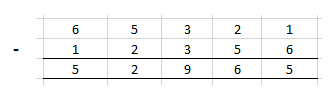= 65321 – 12356

= 52965

9. A machine manufactures 5782 screws every day. How many screws will it manufacture in the month of April?

Solution:

Number of screws manufactured in a day = 5782

Number of days in April = 30 days

Number of screws manufactured in April = 5782 × 30

= 173460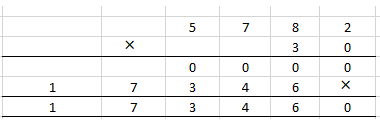Hence, a machine manufactures 173460 screws in the month of April

10. A man has ₹ 157184 with him. He placed an order for purchasing 80 articles at ₹ 125 each. How much money will remain with him after the purchase?

Solution:

A man has = ₹ 157184

Number of articles purchased by him = 80

Cost of one article = ₹ 125

Cost of 80 articles will be = 80 × 125

= ₹ 10000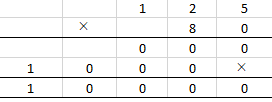Remaining money with him after the purchase = Money in hand – Cost of 80 articles

= 157184 – 10000

= 147184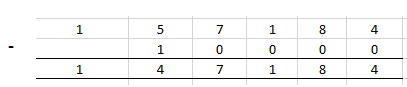11. A student multiplied 8035 by 87 instead of multiplying by 78. By how much was his answer greater than or less than the correct answer?

Solution:

Correct answer = 8035 × 87

= 699045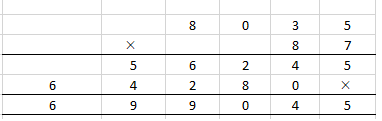Wrong answer = 8035 × 78

= 626730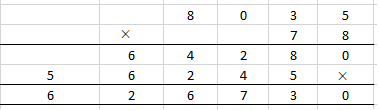Hence, correct answer greater than the wrong answer by = 699045 – 626730

= 72315

12. Mohani has 30 m cloth and she wants to make some shirts for her son. If each shirt requires 2 m 30 cm cloth, how many shirts, in all, can be made and how much length of cloth will be left?

Solution:

Total length of cloth available with Mohani = 30 m

= 30 × 100

= 3000 cm

Each shirt requires = 2 m 30 cm

= 200 cm + 30 cm

= 230 cm cloth

Shirts made by using 30 m cloth = (1 / 230) × 3000

= 13.04

= 13 shirts

Cloth required to make 13 shirts = 230 × 13

= 2990 cm or 29 m 90 cm

∴ Remaining cloth = 30 m – 29 m

= 1 m

13. The weight of a box is 4 kg 800 gm. What is the total weight of 150 such boxes?

Solution:

Weight of a box = 4 kg 800 gm

Total weight of 150 such boxes = 4 kg 800 g × 150

= 720 kg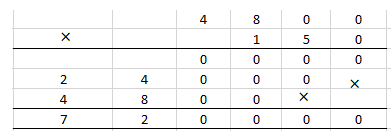Hence, the total weight of 150 such boxes is 720 kg

14. The distance between two places A and B is 3 km 760 m. A boy travels from A to B and then B to A every day. How much distance does he travel in 8 days?

Solution:

Distance between two places A and B = 3 km 760 m

= 3760 m

Distance between A to B and B to A = 2 × 3760 m

= 7520 m

Distance travelled by a boy in 8 days = 7520 × 8

= 60160 m

= 60.16 km

15. An oil-tin contains 6 litre and 60 ml oil. How many identical bottles can this oil fill, if capacity of each bottle is 30 ml?

Solution:

An oil-tin contains = 6 litre 60 ml

= 6 × 1000 + 60

= 6060 ml

Capacity of each bottle = 30 ml

Number of bottles can be filled with 30 ml oil = 6060 ÷ 30

= 202 bottles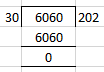## Access another exercise of Selina Solutions Concise Mathematics Class 6 Chapter 1: Number System

Exercise 1(A) Solutions